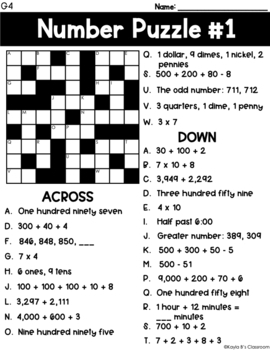# Cross Number Puzzles: Grade 4 (Digital Puzzles Included)Subject
Resource Type
File Type
Zip (1 MB|40 pages)
Standards
\$5.00
• Product Description
• Standards

In this product, you will find 20 cross number puzzles geared towards 4th grade math standards. The puzzles increase in difficulty through the set. However, all the puzzles heavily emphasize mental math strategies, place value knowledge and the ability to structure numbers to 10 and 20. These puzzles are great for homework, small group work, math rotations, intervention or early finishers.

***Digital Version Now Included - Using Google Slides***

When you download, you will find a PDF with clickable links for each puzzle that has been converted into a Google Slide. Each link will force you to make a copy of that file to add to your own personal Google Drive. When assigned to students, they will simply have to click each box and type in their answer.

Puzzles 1-10 Skills:

• place value using base 10 numerals, expanded form and number names

• money

• multiplication

• 1 step patterns

• measurement

• time

Puzzles 11-20 Skills:

• continued practice from previous skills

• multi by single digit multiplication

• double digit multiplication

• verbal statements

• equations with multiple signs

Answer keys are provided for each number puzzle.

For more Cross Number Puzzles:

Add and subtract mixed numbers with like denominators, e.g., by replacing each mixed number with an equivalent fraction, and/or by using properties of operations and the relationship between addition and subtraction.
Understand addition and subtraction of fractions as joining and separating parts referring to the same whole.
Find whole-number quotients and remainders with up to four-digit dividends and one-digit divisors, using strategies based on place value, the properties of operations, and/or the relationship between multiplication and division. Illustrate and explain the calculation by using equations, rectangular arrays, and/or area models.
Multiply a whole number of up to four digits by a one-digit whole number, and multiply two two-digit numbers, using strategies based on place value and the properties of operations. Illustrate and explain the calculation by using equations, rectangular arrays, and/or area models.
Fluently add and subtract multi-digit whole numbers using the standard algorithm.
Total Pages
40 pages